Finding Value of trignometric functions, given angle

Chapter 3 Class 11 Trigonometric Functions
Concept wise
 sin (π/2 – x) = cos x cos (π/2 – x) = sin x sin (π/2 + x) = cos x cos (π/2 + x) = – sin x sin (3π/2 – x)  = – cos x cos (3π/2 – x)  = – sin x sin (3π/2 + x) = – cos x cos (3π/2 + x) = sin x sin (π – x) = sin x cos (π – x) = – cos x sin (π + x) = – sin x cos (π + x) = – cos x sin (2π – x) = – sin x cos (2π – x) = cos x sin (2π + x) = sin x cos (2π + x) = cos x

### Let us learn how to find and remember these values

1. If the angle is multiple of π/2, i.e. π/2, 3π/2, 5π/2,

then

sin becomes cos

cos becomes sin

If the angle is multiple of π, i.e. π, 2π, 3π,

then

sin remains sin

cos remains sin

2.The sign depends on the quadrant angle is in.

## sin ( π /2 – x)

Since it is π/2,

sin will become cos

Here x is an acute angle

So, π/2 – x = 90 – x is an angle in 1st quadrant.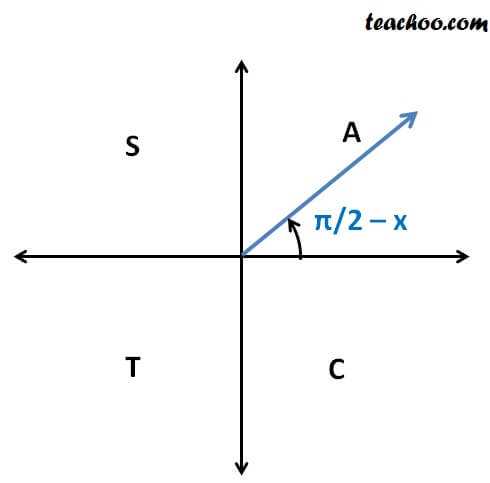Since sin is positive in 1st quadrant

So, sign will be positive

∴ sin (π/2 – x) = cos x

## cos ( π /2 – x)

Since it is π/2,

cos will become sin

Here x is an acute angle

So, π/2 – x = 90 – x is an angle in 1st quadrant.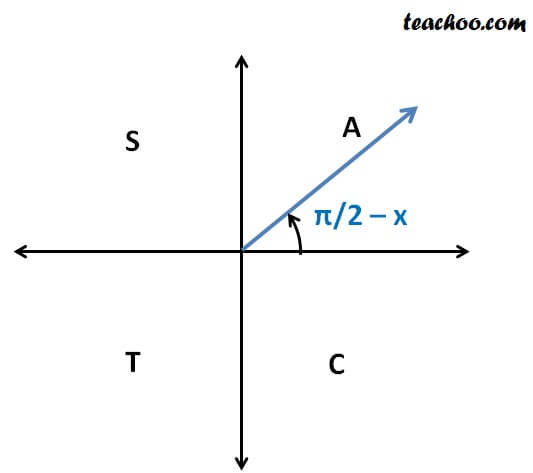Since cos is positive in 1st quadrant

So, sign will be positive

∴ cos (π/2 – x) = sin x

## sin ( π /2 + x)

Since it is π/2,

sin will become cos

Here x is an acute angle

So, π/2 + x = 90 + x

90 + x is an angle which is greater than 90°, less than 180°

So, it will be in 2nd quadrant.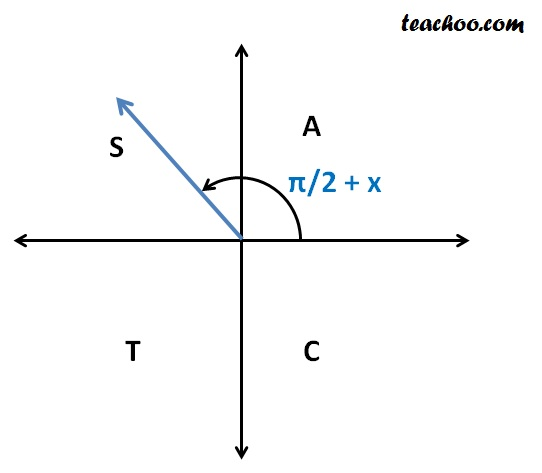Since sin is positive in 2nd quadrant

So, sign will be positive

∴ sin (π/2 + x) = cos x

## cos ( π /2 + x)

Since it is π/2,

cos will become sin

Here x is an acute angle

So, π/2 + x = 90 + x

90 + x is an angle which is greater than 90°, less than 180°

So, it will be in 2nd quadrant.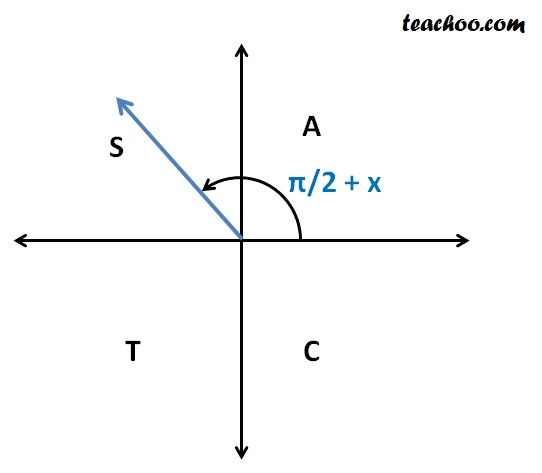Since cos is negative in 2nd quadrant

So, sign will be negative

∴ cos (π/2 + x) = – sin x

## sin (3 π /2 – x)

Since it is 3π/2,

sin will become cos

Here x is an acute angle

So, 3π/2 – x = 270 – x

270 – x is an angle which is greater than 180°, less than 270°

So, it will be in 3rd quadrant.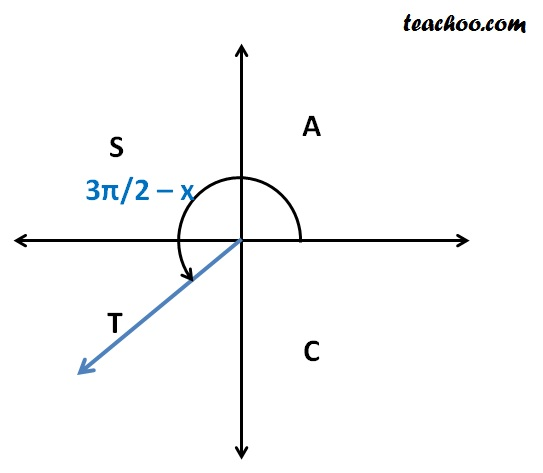Since sin is negative in 3rd quadrant

So, sign will be negative

∴ sin (3π/2 – x) = – cos x

## cos (3 π /2 – x)

Since it is 3π/2,

cos will become sin

Here x is an acute angle

So, 3π/2 – x = 270 – x

270 – x is an angle which is greater than 180°, less than 270°

So, it will be in 3rd quadrant.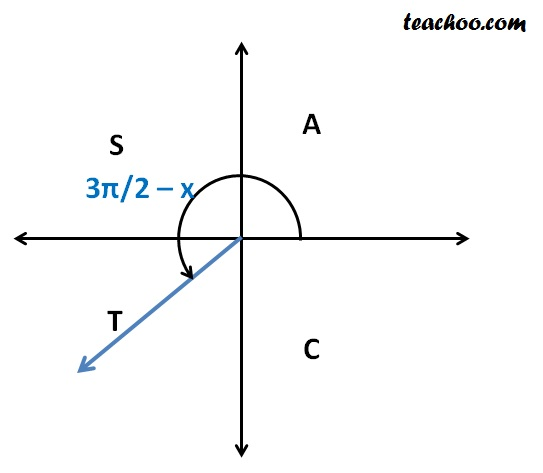Since cos is negative in 3rd quadrant

So, sign will be negative

∴ cos (3π/2 – x) = – sin x

## sin (3 π /2 + x)

Since it is 3π/2,

sin will become cos

Here x is an acute angle

So, 3π/2 + x = 270 + x

270 + x is an angle which is greater than 270°, less than 360°

So, it will be in 4th quadrant.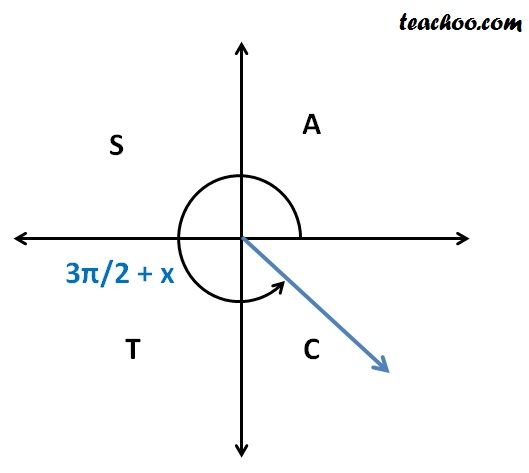Since sin is negative in 4th quadrant

So, sign will be negative

∴ sin (3π/2 + x) = – cos x

## cos (3 π /2 + x)

Since it is 3π/2,

cos will become sin

Here x is an acute angle

So, 3π/2 + x = 270 + x

270 + x is an angle which is greater than 270°, less than 360°

So, it will be in 4th quadrant.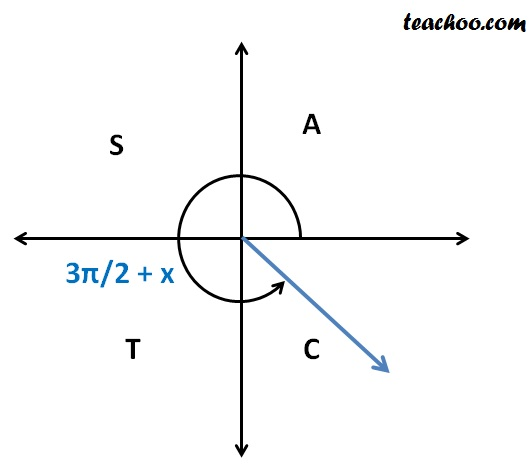Since cos is positive in 4th quadrant

So, sign will be positive

∴ cos (3π/2 + x) = sin x

## sin ( π – x)

Since it is π,

sin will remain sin

Here x is an acute angle

So, π – x = 180 – x

180 – x is an angle which is greater than 90°, less than 180°

So, it will be in 2nd quadrant.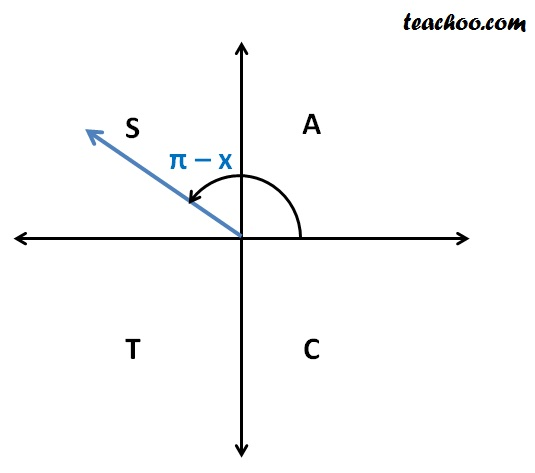Since sin is positive in 2nd quadrant

So, sign will be positive

∴ sin (π – x) = sin x

## cos ( π – x)

Since it is π,

cos will remain cos

Here x is an acute angle

So, π – x = 180 – x

180 – x is an angle which is greater than 90°, less than 180°

So, it will be in 2nd quadrant.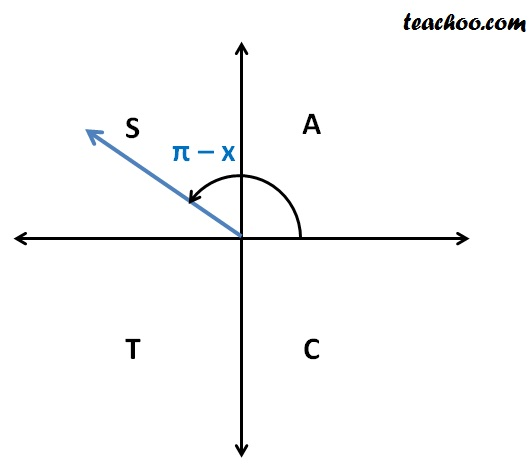Since cos is negative in 2nd quadrant

So, sign will be negative

∴ cos (π – x) = – cos x

## sin ( π + x)

Since it is π,

sin will remain sin

Here x is an acute angle

So, π + x = 180 + x

180 + x is an angle which is greater than 180°, less than 270°

So, it will be in  3rd quadrant.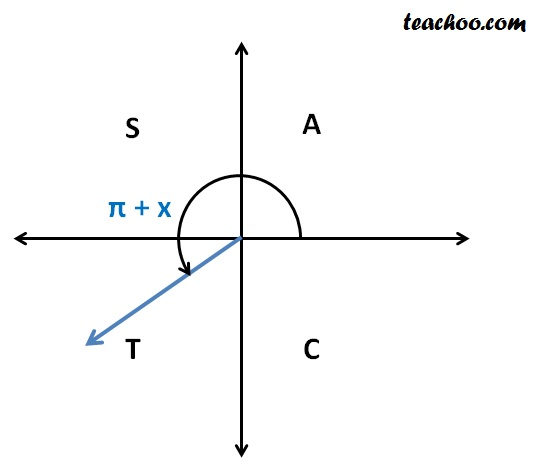Since sin is negative in 3rd quadrant

So, sign will be negative

∴ sin (π + x) = – sin x

## cos ( π + x)

Since it is π,

cos will remain cos

Here x is an acute angle

So, π + x = 180 + x

180 + x is an angle which is greater than 180°, less than 270°

So, it will be in  3rd quadrant.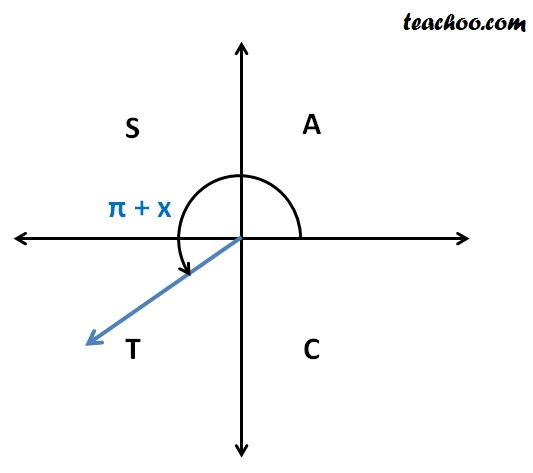Since cos is negative in 3rd quadrant

So, sign will be negative

∴ cos (π + x) = – cos x

## sin (2 π – x)

Since it is 2π,

sin will remain sin

Here x is an acute angle

So, 2π – x = 360 – x

360 – x is an angle which is greater than 270°, less than 360°

So, it will be in  4th quadrant.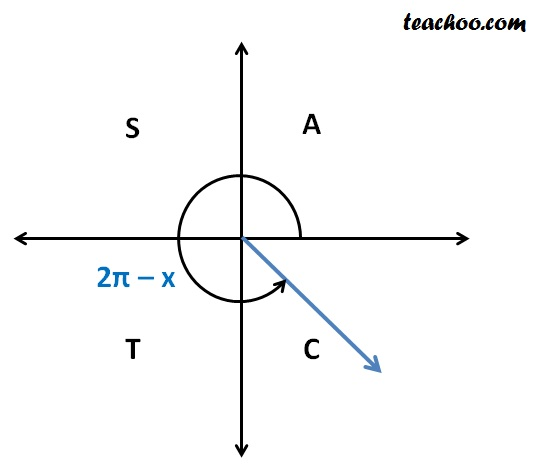Since sin is negative in 4th quadrant

So, sign will be negative

∴ sin (2π – x) = – sin x

We can also prove it by

We know that sin repeats after 2π

⇒ sin (2π – x) = sin (–x)

Since sin (–x) = – sin x

∴ sin (2π – x) = – sin (x)

## cos (2 π – x)

Since it is 2π,

cos will remain cos

Here x is an acute angle

So, 2π – x = 360 – x

360 – x is an angle which is greater than 270°, less than 360°

So, it will be in  4th quadrant.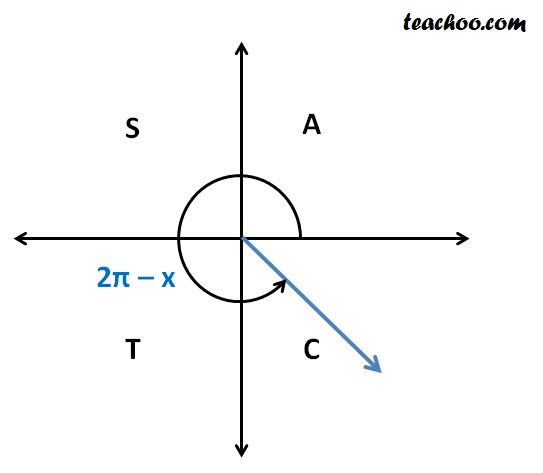Since cos is positive in 4th quadrant

So, sign will be positive

∴ cos (2π – x) = cos x

We can also prove it by

We know that cos repeats after 2π

⇒ cos (2π – x) = cos (–x)

Since cos (–x) = cos x

∴ cos (2π – x) = cos x

## sin (2 π + x)

Since it is 2π,

sin will remain sin

Here x is an acute angle

So, 2π + x = 360 + x

360 – x is an angle which is greater than 360°, less than 360° + 90°

So, it will be in  1st quadrant.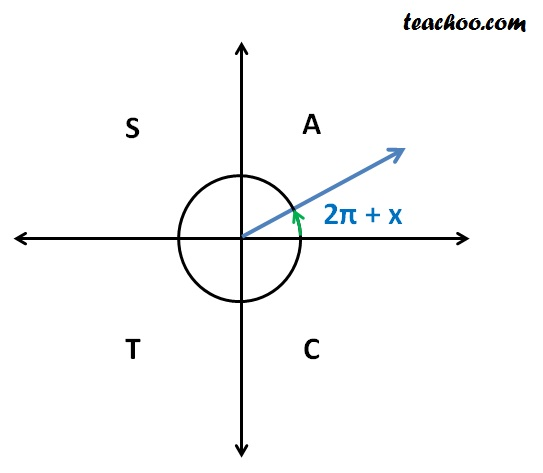Since sin is positive in 4th quadrant

So, sign will be positive

∴ sin (2π + x) = sin x

We can also prove it by

We know that sin repeats after 2π

⇒ sin (2π + x) = sin (x)

## cos (2 π + x)

Since it is 2π,

cos will remain cos

Here x is an acute angle

So, 2π + x = 360 + x

360 – x is an angle which is greater than 360°, less than 360° + 90°

So, it will be in  1st quadrant.Since cos is positive in 4th quadrant

So, sign will be positive

∴ cos (2π + x) = cos x

We can also prove it by

We know that cos repeats after 2π

⇒ cos (2π + x) = cos (x)

Learn in your speed, with individual attention - Teachoo Maths 1-on-1 Class

### Transcript